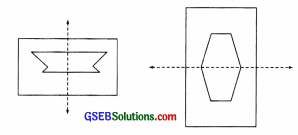# GSEB Solutions Class 6 Maths Chapter 13 Symmetry Ex 13.2

Gujarat Board GSEB Textbook Solutions Class 6 Maths Chapter 13 Symmetry Ex 13.2 Textbook Questions and Answers.

## Gujarat Board Textbook Solutions Class 6 Maths Chapter 13 Symmetry Ex 13.2

Question 1.
Find the number of lines of symmetry for each of the following shapes:Solution:
We find that:
(a) There are four lines of symmetry as shown by dotted lines ‘a’, ‘b’, ‘c’, and ‘d’.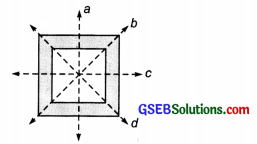(b) There are four lines of symmetry as shown by the dotted lines l1, l2, l3, and l4.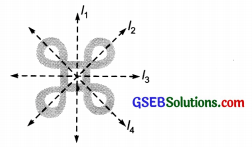(c) There are four lines of symmetry as shown by p, q, r, and s (dotted lines).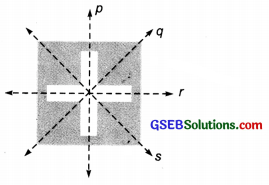(d) The figure has only one line of symmetry as shown by ‘t’ (a dotted line).(e) There are six lines of symmetry as shown by the dotted lines l, m, n, o, p, and q in the figure.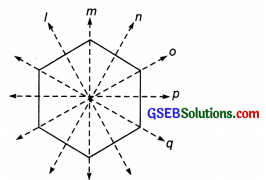(f) In the figure, there are six lines of symmetry as shown by a, b, c, d, e, and f (dotted lines.)(g) The given figure is not symmetrical, therefore it has no line of symmetry.
(h) The given figure is not symmetrical, therefore it has no line of symmetry.
(i) There are four lines of symmetry as shown by the dotted lines p1,p2,p3, and p4.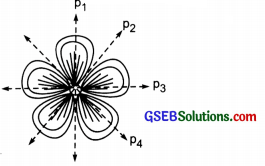Question 2.
Copy the triangle in each of the following figures on squared paper. In each case, draw the line(s) of symmetry, if any, and identify the type of triangle. (Some of you may like to trace the figures and try paper-folding first!).Solution:

(a) The given figure is an equilateral triangle. It has three lines of symmetry as shown by the dotted lines l, m, and n.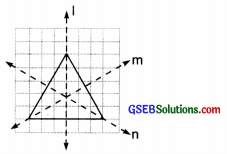(b) The given figure is an isosceles triangle. It has one line of symmetry as shown by the dotted line p. IP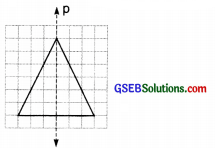(c) The given figure is a right-angled triangle. It has one line of symmetry as shown by the dotted line q.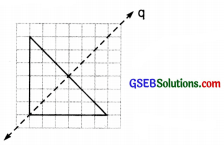(d) The given figure is a scalene triangle which is not a symmetrical figure. Therefore, there is no line of symmetry.Question 3.
Complete the following table.Solution:Question 4.
Can you draw a triangle that has Sketch a rough figure in each case?
(a) exactly one line of symmetry?
Solution:
Yes, an isosceles triangle has exactly one line of symmetry, as shown in the following figure: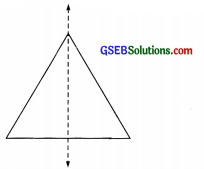(b) exactly two lines of symmetry?
Solution:
No, we cannot draw a triangle having exactly two lines of symmetry.

(c) exactly three lines of symmetry?
Solution:
Yes, an equilateral triangle has exactly three lines of symmetry as shown below:(d) no lines of symmetry?
Solution:
Yes, a scalene triangle has no lines of symmetry.Question 5.
On a squared paper, sketch the following:
Hint: It will be helpful if you first draw the lines of symmetry and then complete the figures.

(a) A triangle with a horizontal line of symmetry but no vertical line of symmetry.
Solution:
An isosceles triangle with a horizontal line of symmetry only is shown in the following figure.(b) A quadrilateral with both horizontal and vertical lines of symmetry.
Solution:
The quadrilateral (rectangle) having both horizontal and vertical lines of symmetry is shown in the following figure.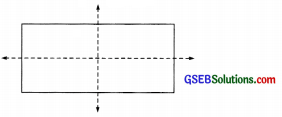(c) A quadrilateral with a horizontal line of symmetry but no vertical line of symmetry.
Solution:
A quadrilateral having only a horizontal line of symmetry is shown in the following figure.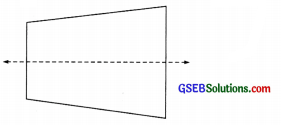(d) A hexagon with exactly two lines of symmetry.
Solution:
A hexagon having exactly two lines of symmetry is shown in the following figure.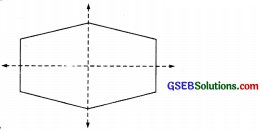(e) A hexagon with six lines of symmetry.
Solution:
A hexagon having six lines of symmetry is shown in the following figure.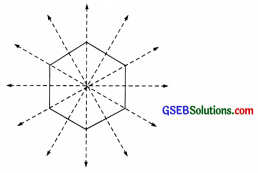Question 6.
Trace each figure and draw the lines of symmetry, if any: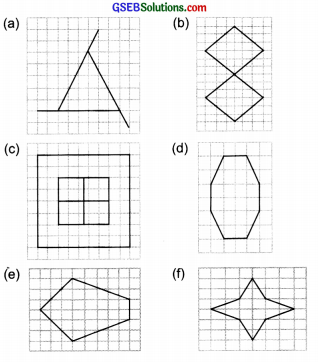Solution:

(a) The given figure is not symmetrical and thus, it has no line of symmetry.(b) The given figure has two lines of symmetry as shown by the dotted lines l and m.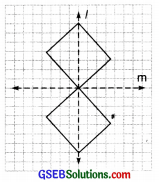(c) The given figure has four lines of symmetry as shown by the dotted lines p,q,r, and s.(d) The given figure has two lines of symmetry as shown by the dotted lines l and m.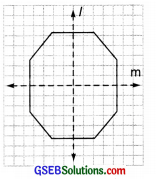(e) The given figure has one line of symmetry as shown by the dotted line ‘l’(f) The given figure has two lines of symmetry as shown in the figure by the dotted lines ‘p’ and ‘q’.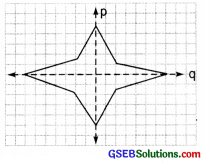Question 7.
Consider the letters of English alphabets, A to Z. List among them the letters which have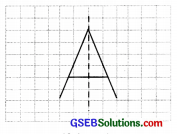(a) vertical lines of symmetry (like A)
Solution:
The following letters are having vertical lines of symmetry: A, H, I, M, O. T, U, V, W, X, and Y.

(b) horizontal lines of symmetry (like B)
Solution:
The following letters are having horizontal lines of symmetry: B, C, D, E, H, I, K, O, and X.

(c) no lines of symmetry (like Q)
Solution:
The following letters are having no lines of symmetry.  G, J, L, N, P, Q, R, S, and Z.

Question 8.
Given here are figures of a few folded sheets and designs drawn about the fold. In each case, draw a rough diagram of the complete figure that would be seen when the design is cut-offSolution:
The complete figure will be seen as given below: about the fold. J In each case,